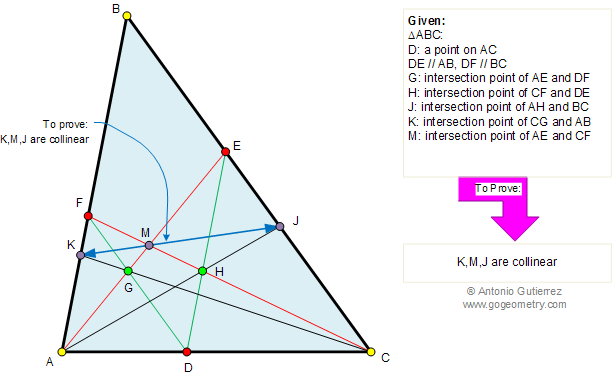Problem 391. Triangle, Parallel lines, Collinear points. Level: High School, SAT Prep, College geometry. The figure shows a triangle ABC with DE parallel to AB and DF parallel to BC. AE intersects DF at G, CF intersects DE at H, AH intersects BC at J, CG intersects AB at K, and AE intersects CF at M. Prove that points K, M, and J are collinear.Recent Additions